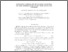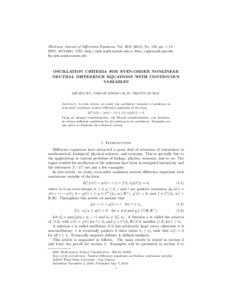# Oscillation criteria for even-order nonlinear neutral difference equations with continuous variables

Wu, Shuhui, Calay, Pargat Singh and Hou, Zhanyuan (2015) Oscillation criteria for even-order nonlinear neutral difference equations with continuous variables. Electronic Journal of Differential Equations, 2015 (128). pp. 1-14. ISSN 1072-6691Preview
Text
Paper_Oscillation criteria.pdf - Accepted Version

Official URL: http://ejde.math.unt.edu/Volumes/2015/128/wu.pdf

## Abstract / Description

In this article, we study the oscillatory behavior of solutions to even-order nonlinear neutral difference equations of the form ∆mτ(x(t) − px(t − r)) + f(t, x(g(t))) = 0. Using an integral transformation, the Riccati transformation, and iteration, we obtain sufficient conditions for all solutions to be oscillatory. Examples are also given to illustrate the obtained criteria.

Item Type: Article 500 Natural Sciences and Mathematics > 510 Mathematics School of Computing and Digital Media Bal Virdee 18 Feb 2019 10:39 18 Feb 2019 10:39 https://repository.londonmet.ac.uk/id/eprint/4622View Item